# KSEEB Solutions for Class 7 Maths Chapter 2 Fractions and Decimals Ex 2.1

Students can Download Chapter 2 Fractions and Decimals Ex 2.1, Question and Answers, Notes Pdf, KSEEB Solutions for Class 7 Maths, Karnataka State Board Solutions help you to revise complete Syllabus and score more marks in your examinations.

## Karnataka State Syllabus Class 7 Maths Chapter 2 Fractions and Decimals Ex 2.1

KSEEB Solutions For Class 7 Maths Chapter 2 Question 1.
Solve:
i)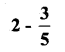Solution: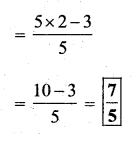ii)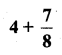Solution: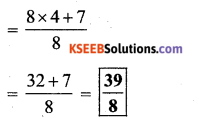iii)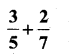Solution: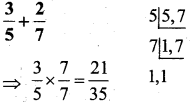L.C.M = 5 × 7 × 1 × 1 = 35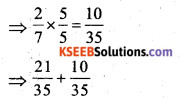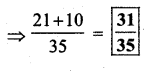iv)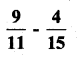Solution:

Online Subtracting Fractions Calculator subtracts the fractions 9/11 and 4/5 i.e. 1/55

The given fractions are 9/11 and 4/5

Firstly the L.C.M should be done for the denominators of the two fractions 9/11 and 4/5

9/114/5

The LCM of 11 and 5 (denominators of the fractions) is 55

Given numbers has no common factors except 1. So, there LCM is their product i.e 55

= 9 x 5 – 4 x 11/55

= 45 – 44/55

= 1/55

Result: 1/55

v)Solution: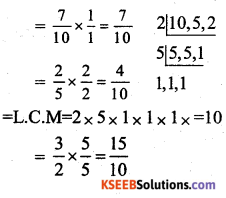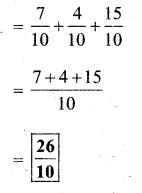vi)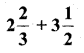⇒ convert mixed fractions to improper fraction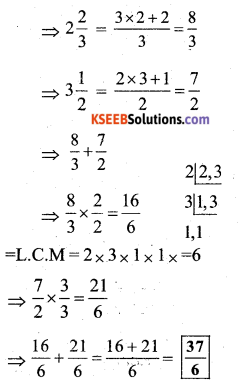vii)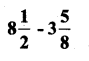convert mixed fractions to improper fraction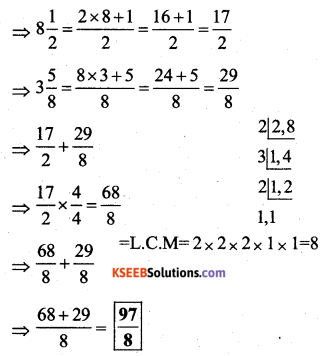KSEEB Solutions For Class 7th Maths Chapter 2 Question 2.
Arrange the following in descending order:
i)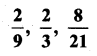= We need to arrange these in descending order,
To find which number is greater or smaller, we make their denominators equal.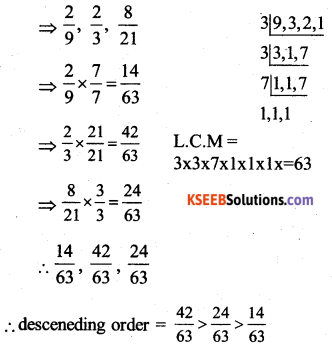ii)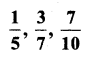⇒ We make their denominators equal, to find the descending order.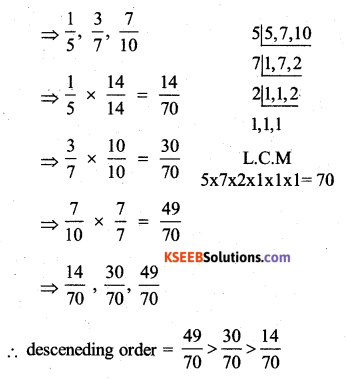KSEEB Solutions For Class 7 Maths Chapter 2 Exercise 2.1 Question 3.
In a “magic square”, the sum of the numbers in each row, in each column and along the diagonals is the same. Is this a magic square ?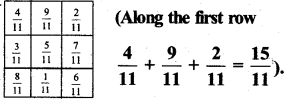Solution:
For Row,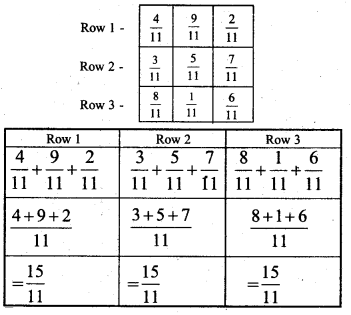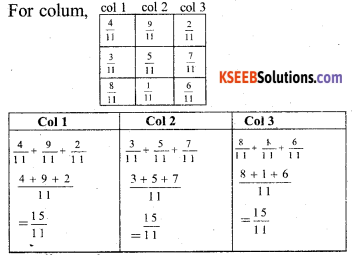For diagonals,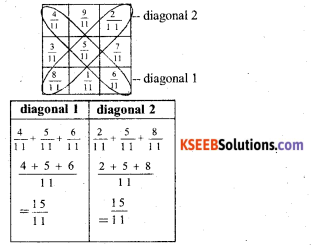Since Sum of air rows, columns and diagonals are equal.KSEEB Solutions For Class 7 Maths Chapter 2 Fractions And Decimals Question 4.
A rectangular sheet of paper is 12$$\frac{1}{2}$$ cm long and 10$$\frac{2}{3}$$ cm wide. Find its perimeter.
Solution:
Length of rectangular sheet of paper = 12$$\frac{1}{2}$$ cm
(breadth) width of rectangular sheet paper = 10$$\frac{2}{3}$$ cm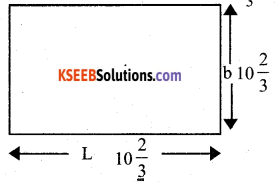Perimeter of rectangle = 2 (length + breadth)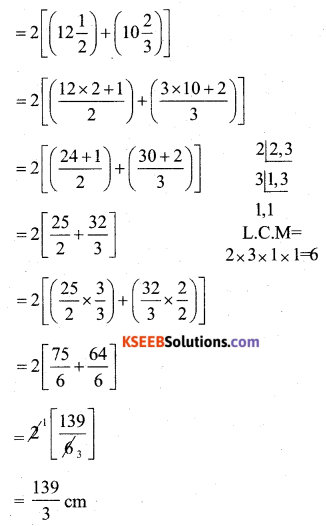converting the above fraction to mixed fraction,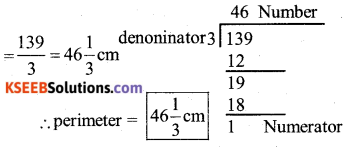KSEEB 7th Maths Solutions Question 5.
Find the perimeters of
(i) ∆ ABE
(ii) the rectangle BCDE in this figure. Whose perimeter is greater ?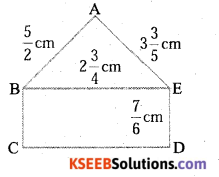Solution:
(i) ∆ ABE
perimeter of ∆ ABE
perimeter = AB + AE + BE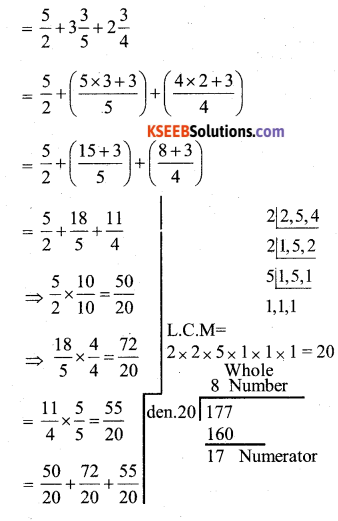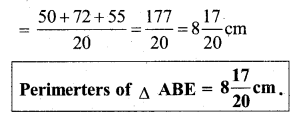ii) The rectangle BCDE in this figure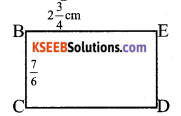Perimeter of rectangle BCDE,
As it is a rectangle, opposite sides are equal
BC = DE CD = BE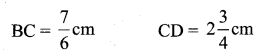perimeter of rectangle = 2(l + b)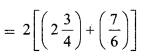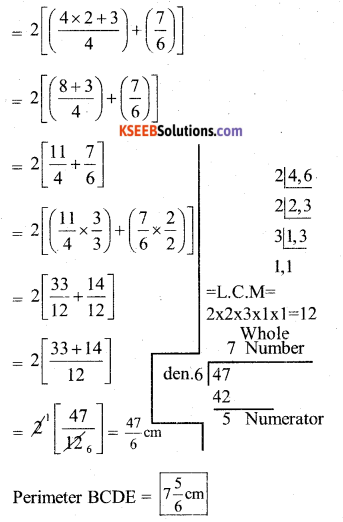Also,
We have to find which perimeter is greater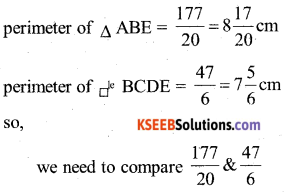To find which fraction is greater, we make its denominator equal.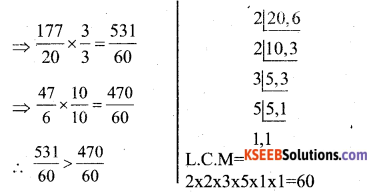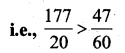Perimeter of ∆ ABE > Perimeter of BCDE
(Thus, Perimeter of ∆ ABE is greater)KSEEB Class 7 Maths Solutions Question 6.
Salil wants to put a picture in a frame. The picture is 7$$\frac{3}{10}$$ cm wide. How much should the picture be trimmed ?
Solution:
There are two things here – picture, and frame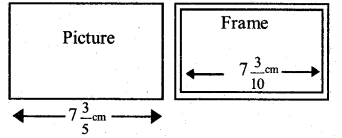so, picture has to be trimmed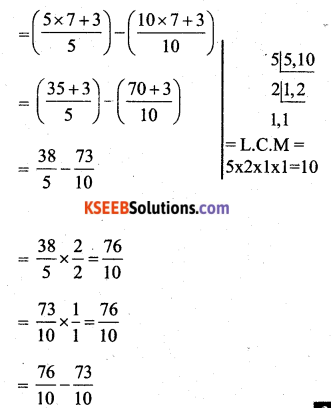$$=\frac{3}{10}$$
∴ Picture has to trimmed by = $$=\frac{3}{10}$$ cm

7th Class Maths Textbook State Syllabus Solutions Question 7.
Ritu ate $$\frac{3}{5}$$ part of an apple and the remaining apple was eaten by her brother Somu. How much part of the apple did Somu eat ? Who had the larger share ? By how much ?
Solution:
Total part = 1
Part eaten by Ritu = $$\frac{3}{5}$$
Part eaten by Somu = Total part – part eaten by Ritu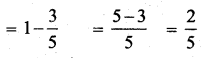Now,
We have to tell who ate the larger share so, we have to compare,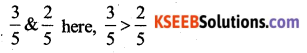∴ Ritu ate the larger share.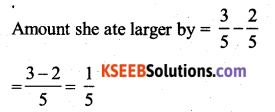Ritu ate large share by $$\frac{1}{5}$$KSEEB Solutions For Class 7th Maths Question 8.
Michael finished colouring in $$\frac{7}{12}$$ hour. Vaibhav finished colouring the same picture in $$\frac{3}{4}$$ hour. Who worked longer ? By what fraction was it longer ?
Solution:
Michael finished work in = $$\frac{7}{12}$$ hour
Vaibhav finished work in = $$\frac{3}{4}$$
We need to find who worked longer.
i.e., we need to find greater of = $$\frac{7}{12}$$ & $$\frac{3}{4}$$
We make their denominators equal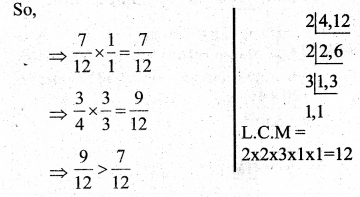∴ Vaibhav worked longer.
We also need to find by how much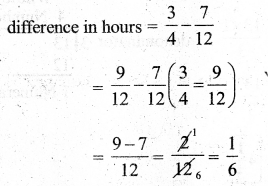Vaibhav worked longer by $$\frac{1}{6}$$ hours.